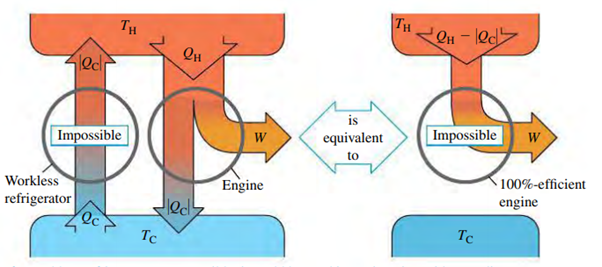Second Law of ThermodynamicsA Qualitative approach

This approach is not an equational approach but rather a statement of impossibility. This includes 'Kelvin-Planck statement' and 'Clausius statement'.

Kelvin-Planck Statement

-‘It is impossible for any system to undergo a process in which it absorbs heat from a reservoir at a single temperature and converts the heat completely into mechanical work, with the system ending in the same state in which it began.’ This statement is also known as Engine statement.Clausius statement

-‘It is impossible for any source to have as its sole result the transfer of heat from a cooler to a hotter body.’ This statement is also known as Refrigerator statement.The above two statements are completely equivalent to each other which is depicted in above Energy diagrams.

A Quantitative approach

This approach uses the concept of Entropy. Entropy provides a quantitative measure of randomness in the system. In an infinitesimal isothermal expansion of an ideal gas , the work done by the gas is equal to the heat added.The fractional volume change dV/V is a measure of the increase in randomness, and it is proportional to quantity dQ/T. The infinitesimal entropy change during an infinitesimal reversible process at absolute temperature T can be written as ,If a total amount of heat Q is added during a reversible isothermal process at absolute temperature T , the total entropy change is given byFor entire process, Entropy change iswhere a is initial state and b is final state.

-Entropy change is independent of the path. A system will have a definite value of entropy that depends only on the state, not on the processes that led to that state.

So, in reverse cyclic process,But  irreversible processes involve an increase in entropy.

Second law of Thermodynamics can be stated as  ‘When changes occur within in a closed system, its entropy either increases for irreversible processes or remains constant for reversible processes. It never decreases.’Microscopic Interpretation of Entropy

When we go deeper, there is microscopic interpretation of Entropy. This interpretation states that for any thermodynamic system, the most probable macroscopic state is the one with the greatest number of corresponding microscopic states, which is also the macroscopic state with the greatest randomness and the greatest entropy. For example, a mole of ideal gas at a certain pressure, volume and temperature , the gas has astronomically large  number of microscopic state depending on the positions and velocities of its molecules equal to Avogadro number. When the gas expands, the number of possible microscopic states increases , becomes more random and the entropy increases.

The entropy in microscopic terms can be calculated as shown below;Where k is Boltzmann constant and w is number of microscopic state for the given macroscopic state.